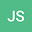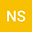Algebraic Lq-norms and complexity-like properties of Jacobi polynomials-Degree and parameter asymptotics
••Nahual Sobrino
DIPC
Author Profile## Abstract

The Jacobi polynomials $\hat{P}_n^{(\alpha,\beta)}(x)$ conform the canonical family of hypergeometric orthogonal polynomials (HOPs) with the two-parameter weight function $(1-x)^\alpha (1+x)^\beta, \alpha,\beta>-1,$ on the interval $[-1,+1]$. The spreading of its associated probability density (i.e., the Rakhmanov density) over the orthogonality support has been quantified, beyond the dispersion measures (moments around the origin, variance), by the algebraic $\mathfrak{L}_{q}$-norms (Shannon and R\’enyi entropies) and the monotonic complexity-like measures of Cram\’er-Rao, Fisher-Shannon and LMC (L\’opez-Ruiz, Mancini and Calbet) types. These quantities, however, have been often determined in an analytically highbrow, non-handy way; specially when the degree or the parameters $(\alpha,\beta)$ are large. In this work, we determine in a simple, compact form the leading term of the entropic and complexity-like properties of the Jacobi polynomials in the two extreme situations: ($n\rightarrow \infty$; fixed $\alpha,\beta$) and ($\alpha\rightarrow \infty$; fixed $n,\beta$). These two asymptotics are relevant \textit{per se} and because they control the physical entropy and complexity measures of the high energy (Rydberg) and high dimensional (pseudoclassical) states of many exactly, conditional exactly and quasi-exactly solvable quantum-mechanical potentials which model numerous atomic and molecular systems.
12 Oct 2021Submitted to International Journal of Quantum Chemistry
18 Oct 2021Submission Checks Completed
18 Oct 2021Assigned to Editor
20 Oct 2021Reviewer(s) Assigned
06 Nov 2021Review(s) Completed, Editorial Evaluation Pending
09 Nov 2021Editorial Decision: Revise Minor